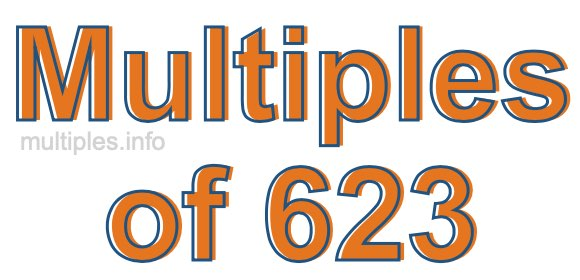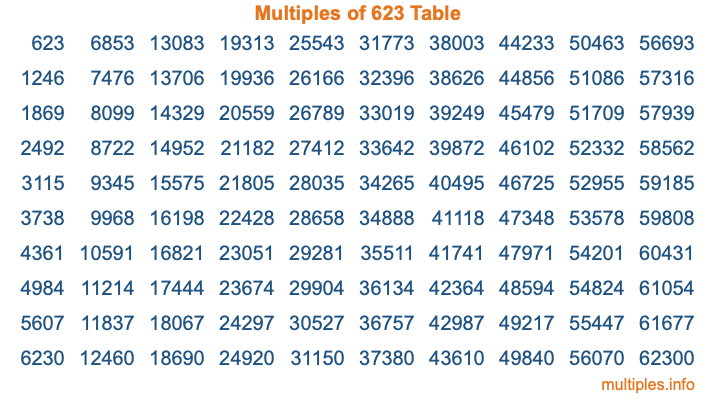Multiples of 623Welcome to the Multiples of 623 page. Here we will first teach you everything you will ever need to know about the multiples of 623, and then give you a study guide summary of everything we taught you to make sure you remember it all. Use this page to look up facts and learn information about the multiples of 623. This page will make you a multiples of six hundred twenty-three expert!

Definition of Multiples of 623
Multiples of 623 are all the numbers that when divided by 623 equal an integer. Each of the multiples of 623 are called a multiple. A multiple of 623 is created by multiplying 623 by an integer.

Therefore, to create a list of multiples of 623, you start with 1 multiplied by 623, then 2 multiplied by 623, then 3 multiplied by 623, and so on for as long as you want. Thus, the list of the first five multiples of 623 is 623, 1246, 1869, 2492, and 3115. To see a larger list of multiples of 623, see the printable image of Multiples of 623 further down on this page. We also have a category where you can choose any nth multiple of 623.

Multiples of 623 Checker
The Multiples of 623 Checker below checks to see if any number of your choice is a multiple of 623. In other words, it checks to see if there is any number (integer) that when multiplied by 623 will equal your number. To do that, we divide your number by 623. If the the quotient is an integer, then your number is a multiple of 623.

Is  a multiple of 623?

Least Common Multiple of 623 and ...
A Least Common Multiple (LCM) is the lowest multiple that two or more numbers have in common. This is also called the smallest common multiple or lowest common multiple and is useful to know when you are adding our subtracting fractions. Enter one or more numbers below (623 is already entered) to find the LCM.

Check out our LCM Calculator if you need more details about the Least Common Multiple or if you need the LCM for different numbers for adding and subtraction fractions.

nth Multiple of 623
As we stated above, 623 is the first multiple of 623, 1246 is the second multiple of 623, 1869 is the third multiple of 623, and so on. Enter a number below to find the nth multiple of 623.

th multiple of 623

Multiples of 623 vs Factors of 623
623 is a multiple of 623 and a factor of 623, but that is where the similarities end. All postive multiples of 623 are 623 or greater than 623. All positive factors of 623 are 623 or less than 623.

Below is the beginning list of multiples of 623 and the factors of 623 so you can compare:

Multiples of 623: 623, 1246, 1869, 2492, 3115, etc.

Factors of 623: 1, 7, 89, 623

As you can see, the multiples of 623 are all the numbers that you can divide by 623 to get a whole number. The factors of 623, on the other hand, are all the whole numbers that you can multiply by another whole number to get 623.

It's also interesting to note that if a number (x) is a factor of 623, then 623 will also be a multiple of that number (x).

Multiples of 623 vs Divisors of 623
The divisors of 623 are all the integers that 623 can be divided by evenly. Below is a list of the divisors of 623.

Divisors of 623: 1, 7, 89, 623

The interesting thing to note here is that if you take any multiple of 623 and divide it by a divisor of 623, you will see that the quotient is an integer.

Multiples of 623 Table
Below is an image of the first 100 multiples of 623 in a table. The table is in chronological order, column by column. The first column has the first ten multiples of 623, the second column has the next ten multiples of 623, and so on.The Multiples of 623 Table is also referred to as the 623 Times Table or Times Table of 623. You are welcome to print out our table for your studies.

Negative Multiples of 623
Although not often discussed or needed in math, it is worth mentioning that you can make a list of negative multiples of 623 by multiplying 623 by -1, then by -2, then by -3, and so on, to get the following list of negative multiples of 623:

-623, -1246, -1869, -2492, -3115, etc.

Multiples of 623 Summary
Below is a summary of important Multiples of 623 facts that we have discussed on this page. To retain the knowledge on this page, we recommend that you read through the summary and explain to yourself or a study partner why they hold true.

There are an infinite number of multiples of 623.

A multiple of 623 divided by 623 will equal a whole number.

623 divided by a factor of 623 equals a divisor of 623.

The nth multiple of 623 is n times 623.

The largest factor of 623 is equal to the first positive multiple of 623.

623 is a multiple of every factor of 623.

623 is a multiple of 623.

A multiple of 623 divided by a divisor of 623 equals an integer.

623 divided by a divisor of 623 equals a factor of 623.

Any integer times 623 will equal a multiple of 623.

Multiples of a Number
Here you can get the multiples of another number, all with the same attention to detail as we did for multiples of 623 on this page.

Multiples of
Multiples of 624
Did you find our page about multiples of six hundred twenty-three educational? Do you want more knowledge? Check out the multiples of the next number on our list!